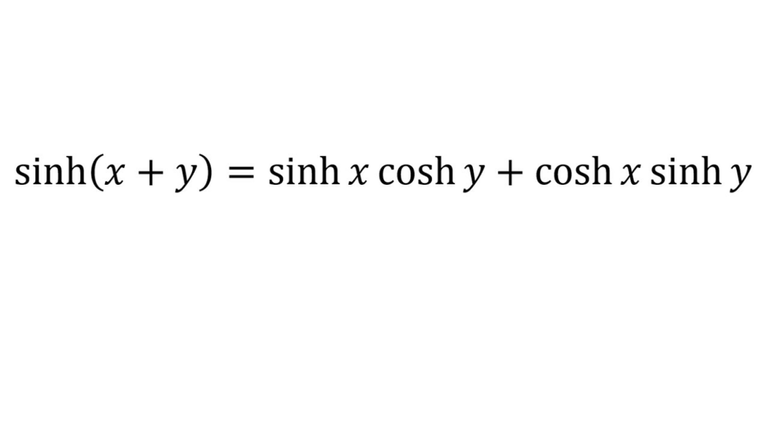# Hyperbolic Trigonometric Identity: sinh(x+y)

in Threespeak2 months ago (edited)In this video I go over the derivation for the hyperbolic trig identity sinh(x + y) = sinh(x)cosh(y) + cosh(x)sinh(y). This derivation is very similar to the one I did for cosh(x + y) in that I show how we need to think of the final result we want before we start the derivation. In other words we need to use the definition of cosh and sinh by multiplying them together to obtain sinh(x+y). Note though that in my earlier video several years back I derived this same identity but by simply working backwards proving the given identity. But in this video I work from start to finish to show how we sometimes have to think outside of the box instead of the typical linear thinking derivations; so make sure to watch this video!

Download the notes in my video: https://1drv.ms/b/s!As32ynv0LoaIhvwZUa6EG1pWW-hq7Q

View video notes on the Hive blockchain: https://peakd.com/mathematics/@mes/video-notes-hyperbolic-trigonometric-identity-sinh-x-y

Related Videos:

Hyperbolic Trigonometric Identity: cosh(x-y): https://youtu.be/GMGaQTym4d4
Hyperbolic Trigonometric Identity: cosh(x+y): https://youtu.be/B_rfrnhx-t4
Hyperbolic Functions - tanh(x), sinh(x), cosh(x) - Introduction: http://youtu.be/EmJKuQBEdlc
Hyperbolic Trigonometry Identity Proof: sinh(x+y) = sinh(x)cosh(y) + cosh(x)sinh(y): http://youtu.be/hrOId-Yp2rk
Hyperbolic Trigonometry Identity Proof: sinh(-x) = -sinh(x), cosh(-x) = cosh(x): http://youtu.be/-Wqq4Wzi7O8 .

SUBSCRIBE via EMAIL: https://mes.fm/subscribe

DONATE! ʕ •ᴥ•ʔ https://mes.fm/donate

Like, Subscribe, Favorite, and Comment Below!

Follow us on:

MES Truth: https://mes.fm/truth
Official Website: https://MES.fm
Hive: https://peakd.com/@mes
Gab: https://gab.ai/matheasysolutions
Minds: https://minds.com/matheasysolutions
Twitter: https://twitter.com/MathEasySolns
Facebook: https://fb.com/MathEasySolutions
LinkedIn: https://mes.fm/linkedin
Pinterest: https://pinterest.com/MathEasySolns
Instagram: https://instagram.com/MathEasySolutions
Email me: [email protected]

Free Calculators: https://mes.fm/calculators

BMI Calculator: https://bmicalculator.mes.fm
Grade Calculator: https://gradecalculator.mes.fm
Mortgage Calculator: https://mortgagecalculator.mes.fm
Percentage Calculator: https://percentagecalculator.mes.fm

Free Online Tools: https://mes.fm/tools

iPhone and Android Apps: https://mes.fm/mobile-apps

▶️ 3Speak

Sort:

You showed me a really nice solution. I like your trigonometry solution to this problem quite well. Our honors were our maths until the second year and these parts of trigonometry seemed a bit of a hassle to me. But you have explained very simply and beautifully.

Glad to you liked the tutorial!